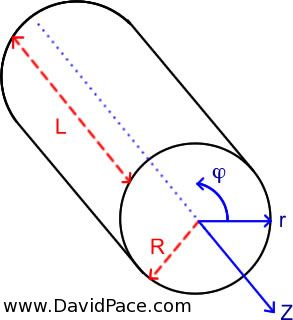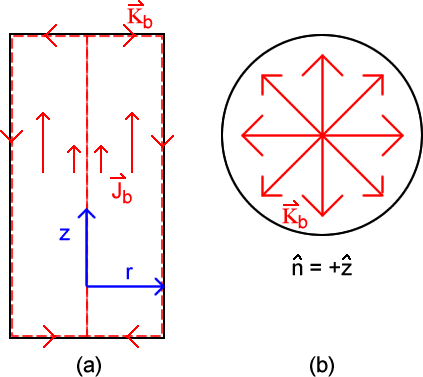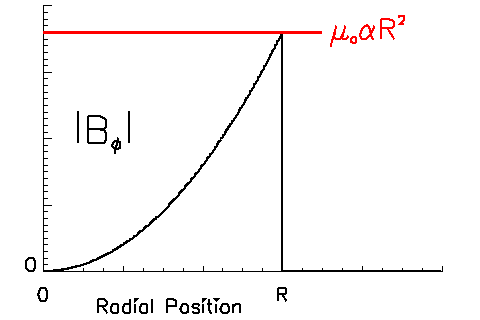# Magnetic Field from a Cylinder’s Magnetization

It is not intuitively obvious how an object’s magnetization relates to the magnetic field it generates. To develop a better physical picture of this phenomena consider an object with a given magnetization and then solve for the resulting magnetic field, B.

A cylinder of radius, R, and length, L, in which R << L (i.e. the cylinder is so long that we will not be concerned with edge effects) is known to have a magnetization, M, given by M = α r2 Φ, where α is a constant. Figure 1 shows this cylinder. Note that the drawing does not accurately reflect that the cylinder is much longer than it is wide.

The goal is to determine the magnetic field of this object. In general, magnetic fields are determined according to the kind of source we expect. Currents are one known source for magnetic field, while time dependent electric fields are another. In this case, the magnetization of an object is known to be related to currents (bound currents to be precise) and there is no mention of any time dependence so it is sensible to proceed with the intention of solving for the magnetic field using currents as a source. Once the currents are known, the magnetic field may be calculated using Ampere’s law,$\oint \vec{B} \cdot d\vec{l} = \mu_oI_{enc}$

where the integral is taken over the entire closed loop of length, l, μo = 4π × 10-7 H m-1 is the permeability of free space, and Ienc is the current enclosed by the loop, l.

The magnetization depends on the radial coordinate of the cylinder. At the position, r = 0, the magnetization is zero. Magnetization is a property of the material, so it is also equal to zero outside of the cylinder (if there is no cylinder, then there cannot be any magnetization). We should now realize that the currents must be determined in two different regions; inside the cylinder and outside of the cylinder. Building on this intuition we should also expect that the magnetic field must be determined as a function of space both inside and outside of the cylinder.Figure 1: The very long cylinder of this example. Note that R is much less than L, although that is not reflected in the drawing (to show that property in the drawing would require drawing a line).

Magnetization is related to bound currents. Since the cylinder has a magnetization throughout its interior, both a bound volume current density and a bound surface current density must be examined. The bound volume current density, Jb, is related to the magnetization by, Jb = × M. The bound surface current density is related to the magnetization by Kb = M × n, where n is the unit vector that is normal to the surface being investigated (it is equivalent to n-hat as seen in the equations that are presented as images).

Begin by solving for the bound volume current density. In the region outside of the cylinder, r > R, the magnetization is zero and therefore, Jb = 0. Inside the cylinder we have,$\vec{J}_b = \vec{\nabla}\times\alpha r^2\hat{\phi}$$\vec{J}_b= \frac{1}{r} \left[ \frac{\partial}{\partial r}\left( r \cdot \alpha r^2\right)\right] \hat{z}$$\vec{J}_b= \frac{\alpha}{r} \left( \frac{\partial}{\partial r} r^3\right)\hat{z}$$\vec{J}_b= \frac{\alpha}{r}\left( 3r^2\right)\hat{z}$$\vec{J}_b= 3\alpha r\hat{z}$

Next, move on to the bound surface current. There are three surfaces of the cylinder to evaluate; the tubular surface of length, L, and the two circular faces. The cylinder has been purposefully made very long so that end effects may ignored. We’ll consider the bound surface current densities on the end faces anyway, for the sake of further developing our physical intuition with respect to bound current paths. The circular faces have unit normals of ± z, depending on which end we are looking at figure 1 illustrates this, but be sure to understand that the unit normal to a surface is always directed away from the object). At the end face shown in figure 1 the bound surface current density is given by,$\vec{K}_{b,+z} = \alpha r^2\hat{\phi}\times \hat{z}$$\vec{K}_{b,+z} = \alpha r^2 \hat{r}$

and for the other end face the surface current density is along the –r direction (technically, this assumes that the cylinder is centered on the position (r,z) = (0,0) and that we are examining the face located at z = L / 2, which was not mentioned in the beginning because end effects were said to be unimportant). Once again, this does not matter for determining the magnetic field of this object because we have decided to purposely neglect contributions from the end of the cylinder. The reason for doing this is that the solution will be simpler if we concern ourselves with only the central region of the cylinder. It is important to note that even bound currents form closed loops. Figure 2 provides an illustration of how this is accomplished in the present discussion. Notice that on one end face the bound surface current flows outward from the center while at the other face it flows inward towards the center (r = 0). We know that the bound volume current density increases with radial position and always flows along +z. The red lines in figure 2 represent the bound current and show that this current forms closed loops within the cross sectional plane of the cylinder. Now that this has been mentioned, please ignore the effects of these end faces while we continue to examine the magnetic field of this object.Figure 2: Bound current densities result in closed current loops. The red arrows indicate the bound currents. (a) Cross section of cylinder, (b) circular end face with unit normal in the +z direction.

The bound surface current that matters to us is the one along the cylindrical side of this object. This cylindrical surface is located at r = R and the bound surface current density is given by,$\vec{K}_{b,r=R} = \alpha R^2\hat{\phi}\times\hat{r}$$\vec{K}_{b,r=R} = -\alpha R^2 \hat{z}$

All of the current is flowing along the z axis. Neglecting the edge effects means we may ignore the r axis directed currents at the edge faces. This z axis symmetry allows for a direct application of Ampere’s law. Drawing a circular loop centered on the z axis (r = 0) will enclose some amount of current flowing along the z axis. As we expand this loop in radius, it will enclose more current. Once the loop is larger than the radius of the cylinder (r > R) there is no more current that can be enclosed. The two Amperian loops that must be drawn to determine the magnetic field everywhere in space are one inside the cylinder and one completely outside.

For the loop taken inside the cylinder, only bound volume current is enclosed. Ampere’s law gives,$\oint \vec{B}\cdot d\vec{l} = \mu_o I_{enc}$$\oint^{2\pi}_0 \vec{B} \cdot \left( r d\phi\right)\hat{\phi} = \mu_o\int \vec{J}_b \cdot d\vec{a}$

This is the value of the magnetic field inside the cylinder. The only component of magnetic field inside the cylinder is along the Φ direction. The next step is to increase the size of the Amperian loop until it is larger than the radius of the cylinder. The total enclosed current will include contributions from all of the bound volume current density and the bound surface current located at r = R. The left side of Ampere’s law remains the same as that found previously.

Before performing this calculation, a mention of the bound surface current must be made. When dealing with a bound surface current density, the total bound surface current is found by multiplying this density and the length over which it passes. Surface current density has units of Amperes per length ([A] / [m]), so multiplying this by the length across with the current flows results in a value that has units of current ([A]). The current in question is the bound surface current that is flowing along the z axis. This current is passing over a length that is the circumference of the cylinder. Note that this length is determined according to a line that is everywhere perpendicular to the surface current density. In the lines below, lcir is the circumferential length of the cylinder.$2\pi r B_\phi = \mu_o \left( \vec{J}_b \cdot d\vec{a} + \vec{K}_b l_{cir}\right)$$2\pi r B_\phi = \mu_o \left( \int^R_0 \left( 3\alpha r\right) \hat{z} \cdot r dr d\phi \hat{z} + \left(-\alpha R^2 \right)\left(2\pi R \right) \right)$$2\pi r B_\phi = \mu_o\left( 3\alpha (2\pi) \frac{R^3}{3} - 2\pi \alpha R^3 \right)$$2\pi r B_\phi = 0$$B_\phi = 0$

Outside the cylinder there is no magnetic field. The magnetic field everywhere in space is given by,

$latex \vec{B} = \mu_o\alpha r^2 \hat{\phi} \qquad \text{for} 0 < r < R &s=2$ $latex \vec{B} = 0 \qquad \text{for} r > R&s=2$

This topic shows that the magnetization of an object is related to the magnetic field the object generates according to the bound currents. Magnetization is a concept that exists to make it easier to describe bound currents in one term. Imagine how difficult it would be to describe the bound currents throughout the cylinder’s interior and along its surface. Instead of that, we define the magnetization and then leave it to the interested party to solve for the equivalent bound currents. Once these currents are known, the magnetic field is found using techniques common to the current/magnetic field relationship.

The magnitude of the magnetic field is plotted in figure 3.Figure 3: Plot of the angular component of the magnetic field of this cylinder. While inside the cylinder (r less than R) the magnetic field, which has only an angular component, increases as the square of the radial position. Outside of the cylinder there is no magnetic field.

This plot was generated with the following IDL code,


r = FINDGEN( 1e3 ) / 1e3
a = r
cylr = 600
a[ cylr : 999 ] = 0
plomake, r, a^2, yr = [ 0., 0.4 ], ys = 4, xs = 4
AXIS, XAXIS = 0, XTICKNAME = ['0', ' ', ' ', 'R', ' ', ' '], COLOR = 0, CHARTHICK = 2, CHARSIZE = 2
AXIS, YAXIS = 0, COLOR = 0, CHARSIZE = 2, CHARTHICK = 2, YTICKNAME = ['0', ' ', ' ', ' ', ' ']
XYOUTS, 0.2, 0.02, 'Radial Position', COLOR = 0, CHARTHICK = 2, CHARSIZE = 2, /NORMAL
PLOTS, [ 0, .7 ], [ .36, .36 ], /DATA, COLOR = 18, THICK = 3  	XYOUTS, .72, .35, '!4l!3!Io!N!4a!3R!E2!N', COLOR = 18,  		CHARTHICK = 2, CHARSIZE = 3, /DATA
XYOUTS, .15, .55, '|B!I!4u!3!N|', /NORMAL, CHARSIZE = 4, CHARTHICK = 2, COLOR = 0
END